# INTRODUCTION TO CHEMICAL ENERGETICS

Energy changes during chemical reactions

Obviously, lots of chemical reactions give out energy as heat. Getting heat by burning a fuel is a simple example, but you will probably have come across lots of others in the lab.

Other reactions need a continuous supply of heat to make them work. Splitting calcium carbonate into calcium oxide and carbon dioxide is a simple example of this.

Any chemical reaction will involve breaking some bonds and making new ones. Energy is needed to break bonds, and is given out when the new bonds are formed. It is very unlikely that these two processes will involve exactly the same amount of energy – and so some energy will either be absorbed or released during a reaction. You will find this discussed in more detail in the page about bond enthalpies.

Simple energy diagrams

A reaction in which heat energy is given off is said to be exothermic.

A reaction in which heat energy is absorbed is said to be endothermic.

You can show this on simple energy diagrams.

For an exothermic change:Notice that in an exothermic change, the products have a lower energy than the reactants. The energy that the system loses is given out as heat. The surroundings warm up.

For an endothermic change: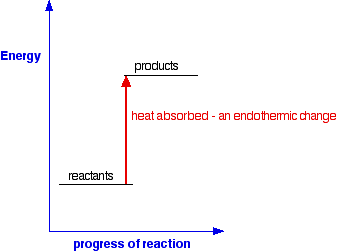This time the products have a higher energy than the reactants. The system absorbs this extra energy as heat from the surroundings.

Expressing exothermic and endothermic changes in numbers

Here is an exothermic reaction, showing the amount of heat evolved: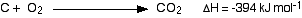This shows that 394 kJ of heat energy are evolved when equation quantities of carbon and oxygen combine to give carbon dioxide. The mol-1 (per mole) refers to the whole equation in mole quantities.

How do you know that heat is evolved? That is shown by the negative sign.

You always think of the energy change during a reaction from the point of view of the reactants. The reactants (carbon and oxygen) have lost energy during the reaction. When you burn carbon in oxygen, that is the energy which is causing the surroundings to get hotter.

And here is an endothermic change: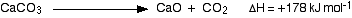In this case, 178 kJ of heat are absorbed when 1 mole of calcium carbonate reacts to give 1 mole of calcium oxide and 1 mole of carbon dioxide.

You can tell that energy is being absorbed because of the plus sign. A simple energy diagram for the reaction looks like this: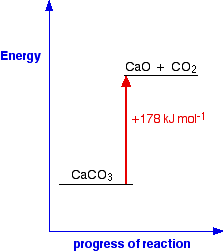The products have a higher energy than the reactants. Energy has been gained by the system – hence the plus sign.

Whenever you write values for any energy change, you must always write a plus or a minus sign in front of it. If you have an endothermic change, and write, say, 178 kJ mol-1 instead of +178 kJ mol-1, you risk losing a mark in an exam.

Energetic stability

You are likely to come across statements that say that something is energetically more stable than something else. For example, in the next page in this section you will find that I have said that oxygen, O2, is more energetically stable than ozone, O3. What does this mean?

If you plot the positions of oxygen and ozone on an energy diagram, it looks like this: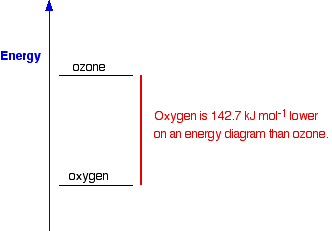The lower down the energy diagram something is, the more energetically stable it is. If ozone converted into ordinary oxygen, heat energy would be released, and the oxygen would be in a more energetically stable form than it was before.

So why doesn’t ozone immediately convert into the more energetically stable oxygen?

Similarly, if you mix petrol (gasoline) and air at ordinary temperatures (when you are filling up a car, for example), why doesn’t it immediately convert into carbon dioxide and water? It would be much more energetically stable if it turned into carbon dioxide and water – you can tell that, because lots of heat is given out when petrol burns in air. But there is no reaction when you mix the two.

For any reaction to happen, bonds have to be broken, and new ones made. Breaking bonds takes energy. There is a minimum amount of energy needed before a reaction can start – activation energy. If the molecules don’t, for example, hit each other with enough energy, then nothing happens. We say that the mixture is kinetically stable, even though it may be energetically unstable with respect to its possible products.

So a petrol and air mixture at ordinary temperatures doesn’t react, even though a lot of energy would be released if the reaction took place. Petrol and air are energetically unstable with respect to carbon dioxide and water – they are much higher up the energy diagram. But a petrol and air mixture is kinetically stable at ordinary temperatures, because the activation energy barrier is too high.

If you expose the mixture to a flame or a spark, then you get a major fire or explosion. The initial flame supplies activation energy. The heat given out by the molecules that react first is more than enough to supply the activation energy for the next molecules to react – and so on.

The moral of all this is that you should be very careful using the word “stable” in chemistry!

article taken  from  chemguide.uk.org